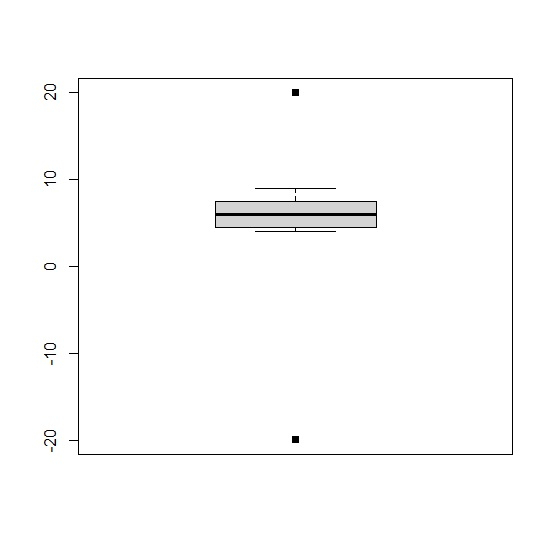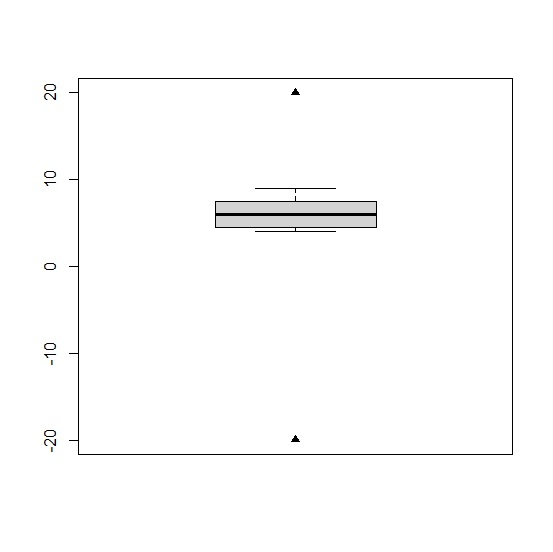# How to display outliers in boxplot with different shape in base R?

To display outliers in boxplot with different shape in base R, we can use outpch argument in boxplot.

For example, if we have a vector called X that contains some outliers then we can create a boxplot of X with different shape of outliers by using the below given command −

boxplot(X,outpch=17)

## Example

To display outliers in boxplot with different shape in base R, use the code given below −

x<-c(-20,rpois(10,5),20)
boxplot(x)

## Output

If you execute the above given code, it generates the following output −To display outliers in boxplot with different shape in base R, use the code given below −

x<-c(-20,rpois(10,5),20)
boxplot(x,outpch=17)

## Output

If you execute the above given code, it generates the following output: −To display outliers in boxplot with different shape in base R, use the code given below −

x<-c(-20,rpois(10,5),20)
boxplot(x,outpch=15)

## Output

If you execute the above given code, it generates the following output −Updated on: 22-Nov-2021

311 Views